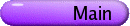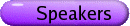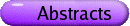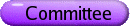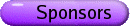Complexity and Computability in Analysis, Geometry, and Dynamics
Org: Alex Nabutovsky and Michael Yampolsky (Toronto)
[PDF]

CARLOS BELTRAN, Universidad de Cantabria, Avda. de Los Castros s/n 39005 Santander, Spain
Advances in homotopy methods for solving systems of complex polynomial equations
[PDF]

Let f be a system of polynomial equations, with complex coefficients. An approximate zero of f is a point z such that the Newton iterates, starting at z, converge quadratically to an exact zero of f. One of the most popular methods to find an approximate zero of f is known as homotopy deformation: Let g be another system which has a known solution z0, and let [g,f] be the segment joining these two systems. Under some (soft) hypotheses, there exists a curve of pairs system-solution Gz0(t) such that Gz0(0) = (g,z0) and Gz0(1) = (f,z), where z is a solution of f. Consider a partition h0 = g,...,hk = f of [g,f], for some positive integer k. Then, Newton's method may be used to (closely) "follow" the curve of solutions Gz0(t), so we obtain a final point zk (close to zk) that may be an approximate zero of the input system f.

The information we need to perform this homotopy deformation is the initial pair (g,z0) and the number of intermediate steps k > 0 (which is the main ingredient for the complexity of the algorithm).

In this talk we will present a probabilistic method designed to find an initial pair (g,z0) satisfying the following, for every e > 0:

A randomly chosen input system f (fixed degrees and number of unknowns) is solved by the homotopy with initial pair (g,z0) and ke steps, with probability 1-e.

Here, ke is a quantity satisfying the following inequality:

 ke £ pe-1,
where p depends polynomially on the size of the input. We conclude that, if we admit a small probability of failure, one can find approximate zeros of systems in polynomial time.

ILIA BINDER, University of Toronto
Computational complexity of conformal maps
[PDF]

We present a memory-efficient algorithm for computing conformal maps. We also discuss the theoretical lower bounds on the computational complexity of the conformal maps to the domains with computable boundaries.

The results were obtained in a joint work with Mark Braverman (University of Toronto) and Michael Yampolsky (University of Toronto).

MARK BRAVERMAN, University of Toronto
Computability and non-Computability of Julia sets
[PDF]

Polynomial Julia sets have emerged as the archetypical examples of invariant fractals generated by complex dynamical systems. Their computer-generated pictures are among the "most drawn" images in mathematics. We are interested in questions of algorithmic computability of these objects.

In our talk we will discuss what it means for a planar compact to be computable, and will present both positive and negative results about the computability of Julia sets.

JOEL HASS, University of California, Department of Mathematics, Davis, CA 95616
Complexity of Knotting
[PDF]

The search for an algorithm to determine if a curve is knotted, first posed by Dehn in 1910, is one of the classical problems of topology. The first algorithm was found by Haken 50 years later. The computational complexity of this problem has been investigated recently in joint work with Lagarias and Pippenger. There has been some additional progress recently and I will survey this work.

PASCAL KOIRAN, Ecole Normale Supérieure de Lyon, France
Decision versus evaluation in algebraic complexity theory
[PDF]

Two main categories of problems are studied in algebraic complexity theory: evaluation problems and decision problems. A typical example of an evaluation problem is the evaluation of the permanent of a matrix. Such problems can be studied within Valiant's framework.

Deciding whether a multivariate polynomial has a real root is a typical example of a decision problem. This problem is NP-complete in the Blum-Shub-Smale model of computation over the real numbers.

In this talk I will present a transfer theorem which shows that if certain evaluation problems are easy, then other decision problems (including the above-mentioned NP-complete problem) are easy too.

Therefore, to show that that P is different from NP over the set of real numbers, one should first be able show that certain evaluation problems are hard.

AVNER MAGEN, University of Toronto
Integrality gaps of SDP for Vertex Cover and relations to l1 embeddability of negative type metrics
[PDF]

We study various SDP formulations for vertex cover by adding different constraints to the standard formulation. We show that vertex cover cannot be approximated better than 2-o(1) even when we add the so-called pentagonal inequality constraints to the standard SDP formulation, en route answering an open question of Karakostas. We further show the surprising fact that by strengthening the SDP with the (intractable) requirement that the metric interpretation of the solution is an l1 metric, we get an exact relaxation (integrality gap is 1), and on the other hand if the solution is arbitrarily close to being l1 embeddable, the integrality gap may be as big as 2-o(1). Finally, inspired by the above findings, we use ideas from the integrality gap construction of Charikar to provide a family of simple examples for negative type metrics that cannot be embedded into l1 with distortion better than 8/7-e. To this end we prove a new isoperimetric inequality for the hypercube.

DONALD MARSHALL, University of Washington, Box 354350, Seattle, WA 98195-4350, USA
Geodesic zippers
[PDF]

E. Sharon and D. Mumford [Internat. J. Comp. Vision 70(2006)] classify 2D shapes using "fingerprints" or conformal weldings. If C is a planar Jordan curve and if f and g are conformal maps from the inside and outside of the unit circle to the inside and outside of C, respectively, then h = f-1 °g is a diffeomorphism of the unit circle and is called a "conformal welding".

We give a (numerical) algorithm for the computation of h from C and for the computation of C from h. The algorithm is elementary, easy to program, as well as fast and accurate in practice.

VOLODYMYR NEKRASHEVYCH, Texas A&M University
Algorithmic aspects of self-similar groups
[PDF]

We will discuss algorithmic problems from the theory of self-similar groups and Thurston maps (post-critically finite branched coverings of the sphere). For instance, we will study the problem of deciding when a given Thurston map is equivalent to a rational function and when two Thurston maps are combinatorially equivalent. Both these problems can be expressed in algebraic terms as questions about the corresponding iterated monodromy groups, which are groups generated by automata.

MARK SAPIR, Vanderbilt University, Nashville, TN
Computational complexity and the Dehn functions of groups
[PDF]

Different kinds of filling functions of groups reflect different kinds of computational complexity of the word problem. For example, the isoperimetric function reflects the time complexity. I am going to talk about recent results (joint with Olshanskii) about isoperimetric functions of groups, relationship with complexity of the word problem and asymptotic properties of groups.

RICHARD SCHWARTZ, Brown, Providence, RI, USA
billiards obtuse and irrational
[PDF]

The triangular billiards problem, which goes back 200 years, asks if every triangular-shaped billiard table has a periodic billiard path. The answer is known to be yes for acute triangles, right triangles, and triangles with angles that are rational multiples of p. For several years, Pat Hooper and I have been developing a computer interface, McBilliards, which explores the existence of periodic billiard paths in triangles. In my talk I will illustrate McBilliards and summarize some of the theorems we have proved, based on experimental output from McBilliards. I hope to also illustrate some self-similarity phenomena in the parameter space, and its connection to Fourier series and Veech surfaces.

YOSEF YOMDIN, The Weizmann Institute of Science, Rehovot 76100, Israel
Fourier Transform of Semi-Algebraic Functions
[PDF]

In various specific problems in Signal Processing it was known for a long time that "simple" signals can be accurately reconstructed from a small number of noisy measurements. Recently a serious general progress has been achieved in this direction, with a development of non-linear reconstruction methods for "sparse" signals. (Sparse signals are representable by linear combinations in a certain basis with a small number of non-zero coefficients.)

It is important also to study completely non-linear signal models. It turns out that the parameters of "simple" such models also can be accurately reconstructed from a small number of noisy measurements. The reconstruction usually leads to nonlinear systems of equations.

We illustrate the main features of the non-linear model reconstruction in an important special case: here the "non-linear models" are semi-algebraic functions, while the "measurements" are certain their Fourier coefficients.

The resulting reconstruction problem turns out to be closely related with some problems in Algebraic Geometry and Differential Equations.